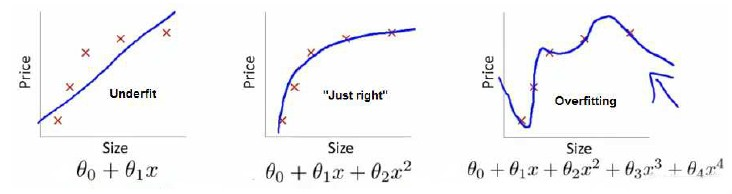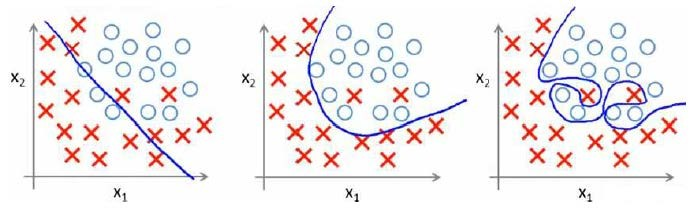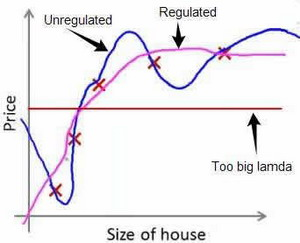# 机器学习--正则化(Regularization)

#### 过拟合问题：没有很好地拟合训练数据，我们称之为欠拟合，或者叫做高偏差


完美的拟合了训练数据，而不能够很好地预测新数据，我们称之为过拟合，或者叫做高方差1. 丢弃一些不能帮助我们正确预测的特征。可以是手工选择保留哪些特征，或者使用一些模型选择的算法来帮忙（例如PCA
2. 正则化。 保留所有的特征，但是减少参数的大小（magnitude）。

### 正则化代价函数

${h_\theta}\left( x \right)={\theta_{0}}+{\theta_{1}}{x_{1}}+{\theta_{2}}{x_{2}^2}+{\theta_{3}}{x_{3}^3}+{\theta_{4}}{x_{4}^4}$

惩罚：就是指减少参数的大小。


$J\left( \theta \right)=\frac{1}{2m}[\sum\limits_{i=1}^{m}{{{({h_\theta}({{x}^{(i)}})-{{y}^{(i)}})}^{2}}+\lambda \sum\limits_{j=1}^{n}{\theta_{j}^{2}}]}$## 正则化逻辑回归模型

${\theta_j}:={\theta_j}-a[\frac{1}{m}\sum\limits_{i=1}^{m}{({h_\theta}({{x}^{(i)}})-{{y}^{(i)}})x_{j}^{\left( i \right)}}+\frac{\lambda }{m}{\theta_j}]$

## 注意：

1. 虽然正则化的逻辑回归中的梯度下降和正则化的线性回归中的表达式看起来一样，但由于两者的${h_\theta}\left( x \right)$不同所以还是有很大差别。
2. ${\theta_{0}}$不参与其中的任何一个正则化。